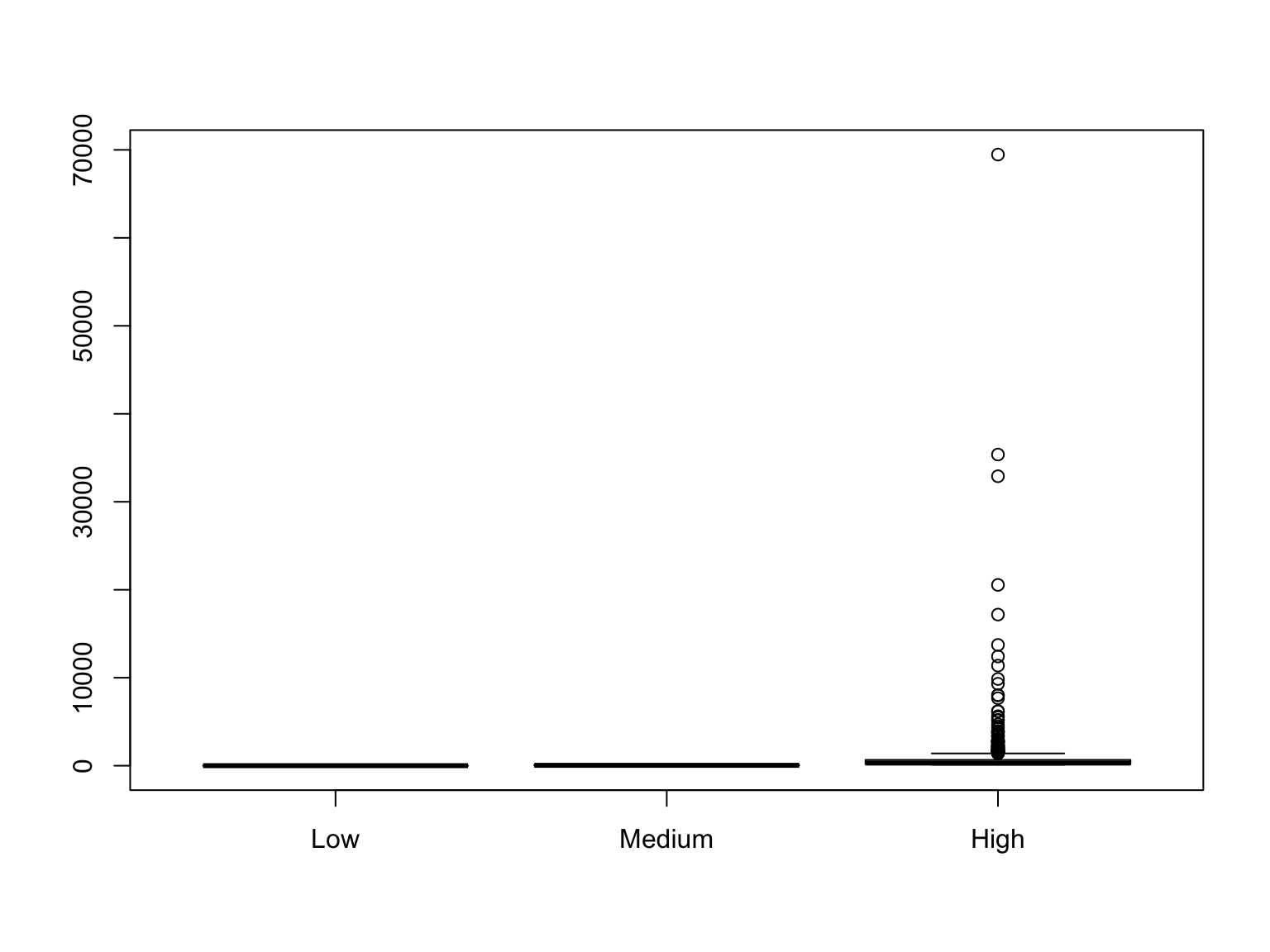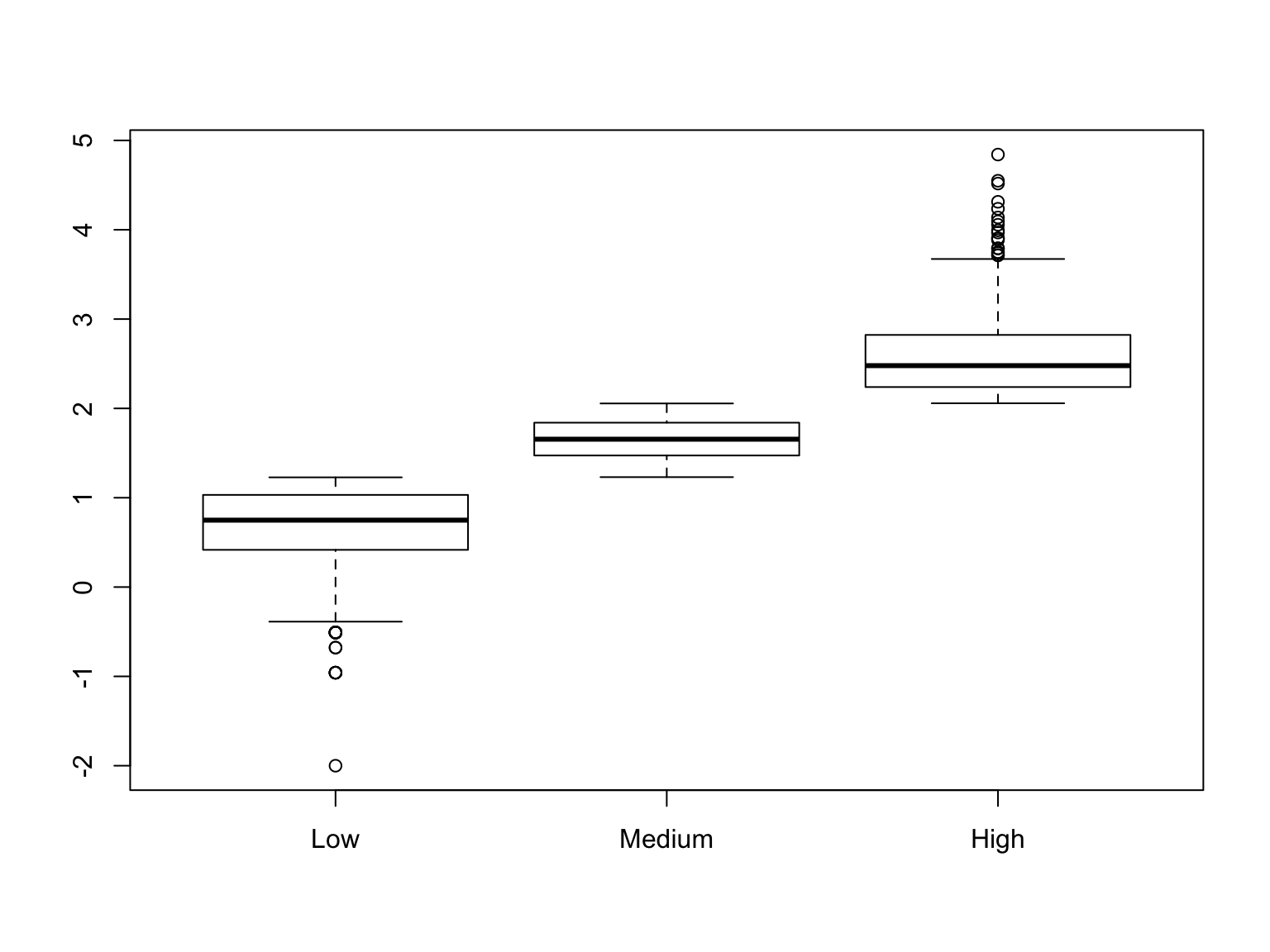11/8/2016

# The Problem Statement

Your task is to create a character variable densityCat in countyComplete with these categorical values. After you create the character variable, you should create a factor version in a second variable densityCatF. Low is defined as the first quartile. Medium is the second and third quartiles. High is the fourth quartile. You will need the quantile function. Use Google for help. It’s your best friend!

Get the data.

cC <- read.delim("~/Dropbox/RProjects/CSC 360 Module 2/countyComplete.txt")

# Solution 1

cC$densityCat = "Low" cC$densityCat[cC$density > quantile(cC$density,.25)] = "Medium"
cC$densityCat[cC$density >
quantile(cC$density,.75)] = "High" cC$densityCatF = factor(cC$densityCat,levels = c("Low","Medium","High")) #Check table(cC$densityCat,cC$densityCatF) ## ## Low Medium High ## High 0 0 786 ## Low 787 0 0 ## Medium 0 1570 0 # Solution 2 cC$densityCatF2 = cut(cC$density, breaks = c(0,quantile(cC$density,.25),
quantile(cC$density,.75), max(cC$density)),
labels=c("Low","Medium","High"),
include.lowest=TRUE)
# Check
table(cC$densityCatF,cC$densityCatF2)
##
##           Low Medium High
##   Low     787      0    0
##   Medium    0   1570    0
##   High      0      0  786

# Let’s Plot

boxplot(cC$density~cC$densityCatF)We have a bad graph because the values have a very large range.

min(cC$density) ##  0 max(cC$density)
##  69467.5

Using Log10 values makes the graph usable.

cC$logDensity = log10(cC$density+.01)
boxplot(cC$logDensity~cC$densityCatF)# Look at Descriptive Statistics by Category

tapply(cC$density,cC$densityCatF,summary)
## $Low ## Min. 1st Qu. Median Mean 3rd Qu. Max. ## 0.00 2.60 5.60 6.83 10.75 16.90 ## ##$Medium
##    Min. 1st Qu.  Median    Mean 3rd Qu.    Max.
##   17.00   29.72   45.20   50.99   69.18  113.60
##
## $High ## Min. 1st Qu. Median Mean 3rd Qu. Max. ## 114.1 173.8 301.1 928.3 664.7 69470.0 tapply(cC$logDensity,cC$densityCatF,summary) ##$Low
##    Min. 1st Qu.  Median    Mean 3rd Qu.    Max.
## -2.0000  0.4166  0.7490  0.6705  1.0320  1.2280
##
## $Medium ## Min. 1st Qu. Median Mean 3rd Qu. Max. ## 1.231 1.473 1.655 1.652 1.840 2.055 ## ##$High
##    Min. 1st Qu.  Median    Mean 3rd Qu.    Max.
##   2.057   2.240   2.479   2.590   2.823   4.842